# 【JavaScript框架封装】使用Prototype给Array，String，Function对象的方法扩充

+关注继续查看

/*
* @Author: 我爱科技论坛
* @Time: 20180705
* @Desc: 实现一个类似于JQuery功能的框架
* V 1.0: 实现了基础框架、事件框架、CSS框架、属性框架、内容框架、动画框架的搭建
* V 2.0：实现了框架的进一步优化，具有良好的扩展性， 可以支持链式访问
* V 4.0: 数据类型的检测、正则表达式的基本用法，数据绑定的知识（模板的使用）
* */

/**
* 用于给js中内置的对象进行扩充方法
*/
(function () {
// 为了使得下面定义的扩充函数执行，祥和里需要调用一下
stringExtend()
arrayExtend()
functionExtend()

// String对象方法的扩充
function stringExtend() {
// str = 'name: @(name), age:@(age)'
// data = {name : 'xiugang', age : 18}
/**
* 实现一个简单的数据绑定
* @param str
* @param data
* @return {*}
*/
String.prototype.formateString = function (data) {
return this.replace(/@$$(\w+)$$/g, function (match, key) {
// 注意这里找到的值必须返回出去(如果是undefined，就是没有数据)
// 注意：判断一个值的类型是不是undefined，可以通过typeof判断
console.log(typeof data[key] === 'undefined');
return data[key] === 'undefined' ? '' : data[key];
});

}
/**
* 去掉坐标的空格
* @param str
* @return {*}
*/
String.prototype.ltrim = function () {
return this.replace(/^\s*/g, '');

}
/**
* 去掉右边的空格
* @param str
* @return {*}
*/
String.prototype.rtrim = function () {
return this.replace(/\s*$/g, ''); } /** * 去掉两边的空格 * @param str * @return {*} */ String.prototype.trim = function () { return this.replace(/(^\s*)|(\s*$)/g, '');
}

// red===>Red
/**
* 将第一个字母小写，其他字母大写
* @param str
* @return {*}
*/
String.prototype.camelCase = function () {
// .*?是非贪婪的匹配，点可以匹配任意字符，星号是前边的字符有0-n个均匹配，问号是则是0-1；
// (^\w{1}): 用于匹配第一个首字母
// (.*)：用于匹配任意个的前面的字符

// - param 1: 匹配到的字符串
// - param 2: 匹配的的子字符串
// - param 3: 匹配的子字符串
// - param 4: 匹配到的字符串在字符串中的位置
// - param 5: 原始字符串

return this.replace(/(^\w{1})(.*)/g, function (match, g1, g2) {
return g1.toUpperCase() + g2.toLowerCase();
});
}
/**
* 将一个字符串的下划线转换为中划线
* @param str
* @return {*}
*/
String.prototype.dashString = function () {
// 这里面的this实际上指向的就是我们自己定义的一个变量字符串
return this.replace(/\_/g, '-');
}

/**
* 检测一个字符串是不是为空
* @return {boolean}
*/
String.prototype.isEmpty = function () {
return this.length === 0;

}
/**
* 判断字符串是不是包含一个字符串
* @param target
* @return {boolean}
*/
String.prototype.contains = function (target) {
// 只要这个indexOf的下标不是-1的话，就说明包含这个目标字符串，否则的话就是不包含
// indexOf() 方法可返回某个指定的字符串值在字符串中首次出现的位置，如果没找到的话，就返回-1
return this.indexOf(target) !== -1;
}
/**
* 对一个字符串中的特殊字符进行转义
* @return {string}
*/
String.prototype.escapeHTML = function () {
/*显示结果	描述	实体名称	实体编号
空格
<	小于号	<	<
>	大于号	>	>
&	和号	&	&
"	引号	"	"
'	撇号 	' (IE不支持)	'
￠	分	¢	¢
�0�5	镑	£	£
�0�6	日圆	¥	¥
€	欧元	&euro
* **/

// 先进行字符串分割， 得到一个数组
var strArr = this.split('');
for (var pos = 0, l = strArr.length, tmp; pos < l; pos++) {
// 拿到数组中的每一个元素
tmp = strArr[pos];
// 对字符串中的每一个元素进行判断， 如果是特殊字符的话就进行处理
switch (tmp) {
// pos始终为1， 表示要替换的项是1项
case '<':
replaceArr(strArr, pos, '<');
break;
case '>':
replaceArr(strArr, pos, '>');
break;
case '\'':
replaceArr(strArr, pos, ''');
break;
case '\"':
replaceArr(strArr, pos, '"');
break;
case '&':
replaceArr(strArr, pos, '&');
break;
default:
;
}
}
// join() 方法用于把数组中的所有元素放入一个字符串。
return strArr.join('');

// 专门用于替换掉数组中的元素
/**
* 替换数组中指定的项
* @param arr
* @param pos
* @param item
* @return {*}
*/
function replaceArr(arr, pos, item) {
// Splice： splice主要用来对JS中的数组进行操作，包括删除，添加，替换等，原来的数组会被改变
// 删除数据：array.splice(index,num)，返回值为删除内容，array为结果值。index为起始项，num为删除元素的的个数。
// 插入数据：array.splice(index,0,insertValue)，index要插入的位置，insertValue要插入的项
// 替换数据：array.splice(index,num,insertValue)，index起始位置，num要被替换的项数，insertValue要替换的值
return arr.splice(pos, 1, item);
}

}
/**
* 忽略HTML中的一些内置的特殊字符
* @return {string}
*/
String.prototype.escapeHTML = function () {
return this.replace(/$/g, '&') .replace(/\</g, '<') .replace(/\>/g, '>') .replace(/\'/g, ''') .replace(/\"/g, '"'); } /** * 对字符串进行反转义 * @return {string} */ String.prototype.unescapeHTML = function () { return this.replace(/&/, '&') .replace(/</g, '<') .replace(/>/g, '>') .replace(/'/g, '\'') .replace(/"/g, '\"') .replace(/&#(\d+)/g, function ($0, $1) { return String.fromCharCode(parseInt($1, 10));
});
}
/**
* 把一个字符串进行反转操作
* @return {string}
*/
String.prototype.reverse = function () {
// 1. 先获得我需要的字符串，然后进行分割处理
var arr = this.toString().split('');
// 2. 对我分割后得到的数组元素进行逆序处理
arr = arr.reverse();
// 3.把数组中的元素变为一个字符串
return arr.join();
//return (this.toString()).split('').reverse().join();
}

}

// Array对象方法的扩充
function arrayExtend() {
/**
* 将一个数组元素清空
* @return {Array}
*/
Array.prototype.clear = function () {
this.length = 0;
return this;
}
/**
* 计算一个数组的长度
* @return {*}
*/
Array.prototype.size = function () {
return this.length;
}
/**
* 返回数组里面的第一个元素
* @return {*}
*/
Array.prototype.first = function () {
return this;
}
/**
* 返回数组的最后一个元素
* @return {*}
*/
Array.prototype.last = function () {
return this[this.length - 1]
}

function cacl(arr, callback) {
// 变量的初始化（治理在使用的时候进行初始化）
var ret;
for (var i = 0, len = arr.length; i < len; i++) {
ret = callback(arr[i], ret);
}
return ret;
}

/**
* 对数组的所有元素进行求和
* @return {*}
*/
Array.prototype.sum = function () {
// 1. 一般的方法
/*var ret = 0;
for (var i = 0, len = this.length; i < len; i++){
ret = ret + this[i];
}
return ret;*/

// 2.使用上面的计算类
/**
* @param:item 数组的每一项
* @param:sum 数组求和的结果
*/
return cacl(this, function (item, sum) {
// 如果刚开始没有初始化的话，就直接使用第一项作为sum（ret）的初始值
if (typeof sum === 'undefined') {
return item;
} else {
return sum += item;
}
})

}
/**
* 找出数组中的最大值
* @return {*}
*/
Array.prototype.max = function () {
// 1. 一般的方式求出最大值
/*var ret = 0;
for (var i = 0, len = this.length; i < len; i++){
if (ret < this[i]){
ret = this[i];
}
}
return ret;*/

// 2. 第二种方式
return cacl(this, function (item, max) {
if (typeof max === 'undefined') {
return item;
} else {
if (max < item) {
return item;
} else {
return max;
}
}
})
}
/**
* 找出一个数组中的最小值
* @return {*}
*/
Array.prototype.min = function () {
return cacl(this, function (item, min) {
if (typeof min === 'undefined') {
return item;
} else {
// 只要每一项的值都不比最小值小的话
if (!(min < item)) {
return item;
} else {
return min;
}
}
})
}

/**
* 求出一个数组中所有元素的平均值
* @return {*}
*/
Array.prototype.avg = function () {
// 1. 先对数组中的元素个数组进行判断一下，防止计算出现无穷的情况
if (this.length === 0) {
return;
}
var sum = this.sum();
return sum / this.length;
/*return cacl(this, function (item, avg) {
// 1. 先求和(进入到这个函数里面， this指向的是window对象，此时window对象是没有sum方法的，故执行错误)
//var sum = this.sum();
// 2.求出平均值
if (typeof avg === 'undefined'){
return item;
} else{
avg = sum / (this.length);
}
return avg;
})*/
}

// 去除数组中的重复项
/*
* 实现思路： 遍历原始数组中的每一项元素，让每次遍历的这一个元素和后面的每一个元素进行比较
* 【只要相同的话就直接跳过继续向下寻找】
* */
Array.prototype.unique = function () {
var a = [],
len = this.length;
for (var i = 0; i < len; i++) {
for (var j = i + 1; j < len; j++) {
if (this[i] === this[j]) {
// 如果找到了相邻的两个元素是相同的，i直接向后移动一位
// 然后j开始从i的位置继续向后寻找元素
j = ++i;
}
}
a.push(this[i]);
}
;
return a;
}
/**
* 去除数组中的重复项
* 【实现思路】：先对数组进行排序，然后比较相邻的元素是否相同
* @return {Array}
*/
Array.prototype.unique = function () {
var tmp = [],
len = this.length;
// 1.先对原始的数组进行排序
this.sort();
// 2.比较相邻的元素
for (var i = 0; i < len; i++) {
// 只要相邻的元素相同，就直接跳过
if (this[i] === this[i + 1]) {
continue;
}

// 由于tmp.length初始的位置一直是0， 添加一个元素之后变为1，因此下标和长度每次相差1， 实现了实时插入数据的功能
tmp[tmp.length] = this[i];
}
return tmp;
}

/**
* 实现两个数组的并集，然后去除重复元素
* @param target
* @return {*}
*/
Array.prototype.union = function (target) {
// concat() 方法用于连接两个或多个数组。
// 连接数组之后然后去除数组中的重复项
return this.concat(target).union();
}

/**
* 求出两个数组的交集
* @param target
* @return {Array|*[]}
*/
Array.prototype.intersect = function (target) {
// 1.先去除原始数组和目标数组中的重复元素
var originArr = this.unique(),
targetArr = target.unique();
// filter()的作用是返回某一数组中满足条件的元素，该方法返回的是一个新的数组
// 2.开始使用条件过滤
/**
* @param element（必选）：当前元素的值
@param index（可选）： 当前元素的索引
@param array（可选）：当前元素所属的数组
*/
return originArr.filter(function (element, index, array) {
// filter函数默认会把所有的返回false的元素去掉
for (var i = 0, len = targetArr.length; i < len; i++) {
if (element === targetArr[i]) {
// 只要是返回满足true的所有条件，基本上都会被过滤掉
return true;
}
//return false;
}
// 只有找到相同的元素的时候返回的是true,其他情况都是返回的是false
return false;
});

}

/**
* 找出两个数组中的不同元素
* @param target
* @return {Array|*[]}
*/
Array.prototype.diff = function (target) {
// 1. 获取原始数组和目标数组，去除重复项
var orignArr = this.unique(),
targetArr = target.unique();
// 2. 开始使用filter函数过滤条件
return orignArr.filter(function (element, index, array) {
for (var i = 0, len = targetArr.length; i < len; i++) {
// 只要元素相等的话，就全部过滤掉
if (element === targetArr[i]) {
return false;
}
}
return true;
});
}

/**
* 对数组的每一项遍历的时候设置一个回调函数（没有返回结果）
* @param fn
* @param ctx
*/
Array.prototype.forEach = function (fn, ctx) {
var i = 0,
len = this.length;
for (; i < len; i++) {
// element, index, array
// call 的第一个参数也就是this的指向， 其他参数表示需要传递给回调函数的的参数
fn.call(ctx || null, this[i], i, this);
}
}
}

/**
*
* 对数组的每一项执行回调，返回由回调函数的结果组成的数组
* @param fn
* @param ctx
* @return {Array}
*/
Array.prototype.map = function (fn, ctx) {
// 初始化变量
var ret = [],
i = 0,
len = this.length;
// 遍历数组的每一项元素， 返回由回调函数的结果组成的数组
for (; i < len; i++) {
// 调用回调函数， 返回指向结果
res = fn.call(ctx || null, this[i], i, this);
// 将每一项执行的结果放入到一个新的数组里面
ret.push(res);
}
return ret;
}
/**
* 对数组的每一项执行回调函数， 返回回调函数执行结果为true的数组集合
* @param fn
* @param ctx
*/
Array.prototype.filter = function (fn, ctx) {
var ret = [],
i = 0,
len = this.length;
// 遍历每一项，把执行结果为true的所有元素集合存起来
for (; i < len; i++) {
// 注意这里的这种运算方式只会返回所有的回调函数返回true的计算结果集
fn.call(ctx || null, this[i], i, this) && ret.push(this[i]);
}
return ret;
}

/**
* 对数组的【每一项】执行回调函数，必须每一项回调函数返回true， 就返回true
* @param fn
* @param ctx
*/
Array.prototype.every = function (fn, ctx) {
var i = 0,
len = this.length;
// 遍历数组中所有的元素， 只要有一个函数回调函数为false就返回false,只要所有的都是true才会返回true
for (; i < len; i++) {
// 如：a默认是undefined，!a是true，!!a则是false，所以b的值是false，而不再是undefined。这样写可以方便后续判断使用。
// 所以，!!(a)的作用是将a强制转换为布尔型（boolean）。
// 如果a = null, !!(a) 的结果就是假， 可以直接把一个弱类型强制转换为一个新的类型
// 下面的代码就是强制将一个函数转换为bool的类型
if(!!fn.call(ctx || null, this[i], i, this) === false)
return false;

// 上面的代码等价于
/*if (fn.call(ctx || null, this[i], i, this)) {
return true;
}*/
}
return true;
}
/**
* 对数组中的每一项执行回调函数，只要有一项为true的话，就是true，否则就是false
* @param fn
* @param ctx
*/
Array.prototype.some = function (fn, ctx) {
var i = 0,
len = this.length;
// 循环遍历每一项，只要有一项为true，就是true
for (; i < len; i++) {
/*
* // 强制转换为Boolean 用 !!
var bool = !!"c";
console.log(typeof bool); // boolean

// 强制转换为Number 用 +
var num = +"1234";
console.log(typeof num); // number

// 强制转换为String 用 ""+
var str = ""+ 1234;
console.log(typeof str); // string
* */
if(!!fn.call(ctx || null, this[i], i, this) === true)
return true;
}
return false;
}

/**
* 从左向右执行回调函数（第二个元素开始）
* 其中包含了上一次回调的返回值
* @param callback
*/
Array.prototype.reduce = function (callback) {
var i = 0,
len = this.length,
callbackRet = this;          // 这个变量保存着上一次回到的函数的返回结果， 默认存储的是第一个元素
for (; i < len; i++) {
// this的指向，element， index， 数组对象本身
// callbackRet 里面存储了数组上一次计算的处理结果
callbackRet = callback.call(null, callbackRet, this[i], i, this);
}
return callbackRet;
}

/**
* 从右向左处理每一项元素，倒数第二项开始执行
* @param callback
*/
Array.prototype.reduceRight = function (callback) {
var len = this.length,
i = this[len - 2],
callbackRet = this[len - 1];        // 保存着最后一项

// 从倒数第二项开始向前遍历数组的每一项
for (; i >= 0; i--) {
//this指向， prev， element, index, arr
callbackRet = callback.call(null, callbackRet, this[i], i, this);
}
return callbackRet;
}

/**
* 返回目标值target在数组中第一次出现的位置， 搜索默认会从左向右执行
* @param target
* @param start
*/
Array.prototype.indexOf = function (target, start) {

/*
* 其实是一种利用符号进行的类型转换,转换成数字类型
~~true == 1
~~false == 0
~~"" == 0
~~[] == 0
~~undefined ==0
~~!undefined == 1
~~null == 0
~~!null == 1
* */
var len = this.length,
start = ~~start;        // 如果start不传过来，这里就是undefined，指向后面的就会保存，这里使用了~~把其他类型强制转换为数字类型
if (start < 0) {
// 如果指定搜索的起始位置小于0的话， 默认就从0的位置开始向后搜索
start = 0;
}
// 从用户指定的起始位置开始向后搜索
for (; start < len; start++) {
if (this[start] === target) {
return start;
}
}
// 如果没找到的话，就返回-1
return -1;
}

/**
* 返回指定的目标值在数组中最后一次出现的位置
* @param target
* @param start
*/
Array.prototype.lastIndexOf = function (target, start) {
// 这里相当于是typeof start ==== 'undefined'
if (start === void 0) {
start = this.length;
} else if (start < 0) {
start = 0;
}

// 开始从数组的最后面向前遍历
for (; start >= 0; start--) {
// 找到目标元素target在数组中最后一次出现的位置（从后向前找）
if (this[start] === target) {
return start;
}
}
return -1;
}

/**
* 数组去重方法加强版本
* 局限性：只适用于数组中存放的是单一的数据类型，如果是多种数据类型并存的话，就会去重失败
* ['ff', 1, '1']
*/
Array.prototype.enhanceUnique = function () {
var ret = [],
tempMap = {},
i = 0,
len = this.length,
temp;

// 遍历数组的每一项
for (; i < len; i++) {
temp = this[i];
// 只要这个tempMap中没有这一项的话，就直接放入到数组中去
if (tempMap[temp] === void 0) {
ret.push(temp);
// {}数据的存储格式为{1 : true, 2 : false, 3 : false}
tempMap[temp] = true;
}
}
return ret;
}

/**
* 删除数组中的指定元素， 通过arguments伪数组的方式来接受传递过来的参数
* 经过测试，只能删除数组中重复的多余的元素
* @return {Array}
*/
Array.prototype.without = function () {
// slice(start, end) 方法可从已有的数组中返回选定的元素。
// 如果slice()这个函数没有指定结束的位置的话，默认是会返回数组中的start之后的所有元素
// 1. 获取用户传过来的参数， 去掉数组中重复的元素
//var args = [].slice.call(arguments).unique();
/*
* Array.prototype.slice.call({
0:"likeke",
1:12,
2:true,
length:3
});
* */
//1. 由于arguments实际上是一个伪数组，不能直接使用数组里面的方法
// 因此先要把arguments转换为数组
var arr = Array.prototype.slice.call(arguments) || [].slice.call(arguments);
// 2. 把数组中的重复元素去重
var args = arr.unique(),
len = this.length,
aLength = args.length,
i = 0,
j = 0;

// 遍历原始的数组(由于后面每次删除掉一个元素之后，这里的this.length的长度就是已经都改变了， 因此每次在执行完毕之后都要重新计算一下length)
for (; i < len; i++) {
for (; j < aLength; j++) {
if (this[i] === args[j]) {
// 只要删除的数组在我的这个里面，就直接去掉
// i 为起始的值，1为要删除的项， 也就是删除i位置的元素
// splice  返回的是删除的元素， this内容是已经修改过之后的项
this.splice(i, 1);

// 为了避免删除数组的元素之后的数组长度的变化，这里需要重新计算一下数组的新的长度
// len = this.length;
}

}
// 将j下标复位，以便下一次循环(注意是在每一次j循环完毕之后然后再把j初始化到原始的状态)
j = 0;
}
return this;
}

/**
* 去掉数组中的目标元素
*/
Array.prototype.enhanceWithout = function () {
// 用于去除数组中指定的的多余的元素
var ret = [],
len = this.length,
args = ([]).slice.call(arguments),
argsLength = args.length,
i = 0,
j = 0;

for (; i < len; i++){
for(; j < argsLength; j++){
if (args[j] !== this[i]){
ret.push(this[i]);
}
}
// 由于这里的j使用的是局部变量，因此这里需要进行处理
j = 0;
}
return ret;
}

/**
* 实现一个数组的扁平化(可以解决数组里面存放数组的问题)【递归处理调用】
* [[], [], [], [[], [], []]]
* @return {Array}
*/
Array.prototype.flatten = function () {
// 实现一个flatten函数，将一个嵌套多层的数组 array（数组） (嵌套可以是任何层数)转换为只有一层的数组
// 数组中元素仅基本类型的元素或数组，
var ret = [],
len = this.length,      // 注意当下一次执行递归调用之后，这里的this指向的是tmp
i = 0,
tmp;

for (; i < len; i++) {
// 注意这里先取出来数组中的每一项元素
tmp = this[i];
// 判断一下数组里面存放的还是不是数组类型（数组里面的每一项）
if (({}).toString.call(tmp) === '[object Array]' || Object.prototype.toString.call(tmp) === '[object Array]') {
// 继续递归调用(递归调用的时候需要把结果存起来哦)
// 1. 对当前数组里面的数组进行扁平化处理, tmp.flatten()得到的就是一个普通的数组类型
// 2. 由于ret是一个数组类型，使用concat之后可以把两个数组里面的元素链接起来
// 下一次执行递归的时候上面的this就是指向了这里的tmp数组
ret = ret.concat(tmp.flatten())
//tmp.flatten();
} else {
// 如果不是数组类型的话，就直接放入到我的新数组里面
ret.push(tmp);
}
}
return ret;
}

/**
* 删除数组中的指定位置的项
* @param pos
* @return {Array}
*/
Array.prototype.removeAt = function (pos) {
// 移出数组中指定位置的项
// slice() 函数调用的执行结果返回的是删除掉的项， 这个this就是修改之后的项
this.splice(pos, 1);
return this;
}

/*
【经验话语1】
直接用等号 （==） 判断时，变量必须要声明（包括不用var 的隐式声明），否则出错。
不管变量有没有声明，都可用typeof 判断，注意typeof 返回结果为字符串，所以是与"undefined"做比较。
所以，判断类型最好用typeof ，因为当判断的变量是在其他js 文件中定义的全局变量时，
执行此判断时，定义该变量所在的js 文件可能还未加载完成，用== 判断就会报错：is not defined
【经验话语2】

注意slice()和splice（） 这两者的区别
* */

/**
* 检测数组中是不是包含某一项
* @param target
* @return {boolean}
*/
Array.prototype.contains = function (target) {
// 可以调用自己之前申明好的some方法，数组中只要有一项，就会返回true
return this.some(function (element, index, self) {
// 调用this.some()方法实际上会返回遍历数组元素的每一项
return element === target;
})
}

/**
* 随机返回数组中的某一项(把数组中的任意一项返回)
* @param n
* @return {*}
*/
Array.prototype.random = function (n) {
//Math.floor():向下取整。Math.floor(1.8) -> 1
//Math.ceil():向上取整。Math.ceil(1.1) -> 2
//v = Math.random() * n:会产生一个 0 < v < nv的数
//v2 = Math.floor(Math.random() * n)：v2为一个大于等于0，小于n的整数
var index = (Math.floor(Math.random()) * n);
return this[index] || this[this.length-1];
}

// Function对象方法的扩充
function functionExtend(func) {
Function.prototype.before = function () {
// 一般来说加下划线的变量为私有变量，这是常规都比较遵守的一种代码规范。
var __self = this;      // 私有的属性用下划线
return function () {
// 重新把我需要传递的参数传递过去， 如果目标函数返回的是false, 就是false
if (func.apply(this, arguments) === false){
return false;
}
// 否则就把我的自己的参数传递过去
return __self.apply(this, arguments);
}
}

/**
* AOP 切面编程的函数扩充
* @param func
* @return {Function}
*/
Function.prototype.after = function (func) {
var __self = this;
return function () {
var ret = __self.apply(this, arguments);        // //返回一个函数，相当于一个代理函数，也就是说，这里包含了原函数和新函数，原函数指的是myFunc，新函数指的是fn
if (ret === false){
return false;
}
func.apply(this, arguments);
return ret;
}
}

}
})();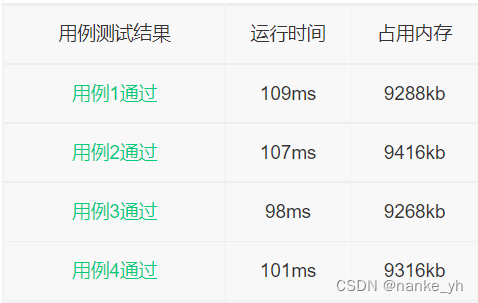0 0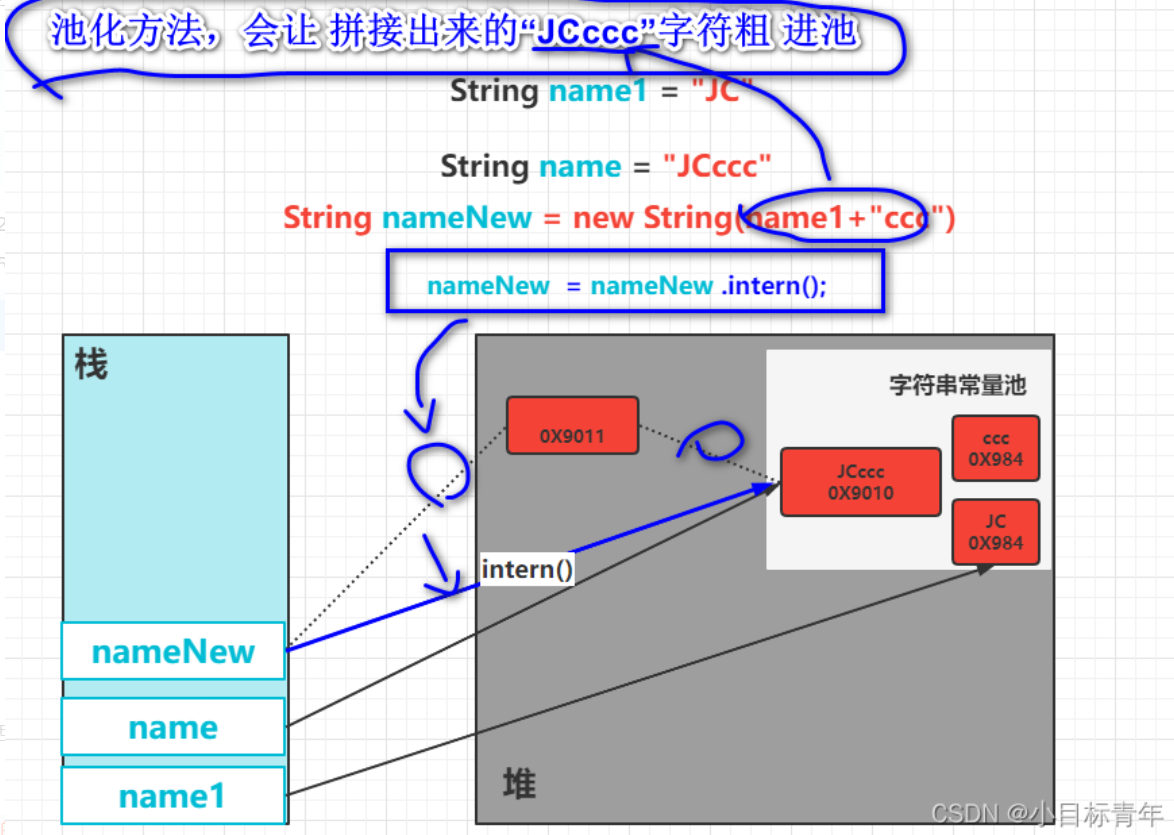Java 面试，创建了几个String 对象？ 我让问！让你问！让你问！(二）
Java 面试，创建了几个String 对象？ 我让问！让你问！让你问！(二）
0 0Java 面试，创建了几个String 对象？ 我让问！让你问！让你问！(一）
Java 面试，创建了几个String 对象？ 我让问！让你问！让你问！(一）
0 0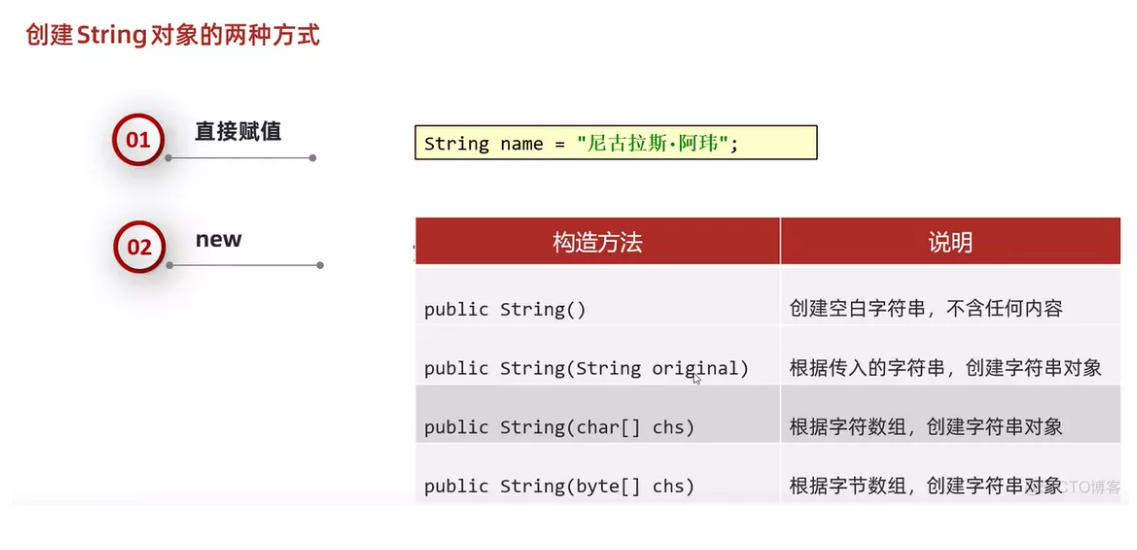java学习第九天笔记-方法176-构造方法代码分析-创建string对象的方式
java学习第九天笔记-方法176-构造方法代码分析-创建string对象的方式
0 0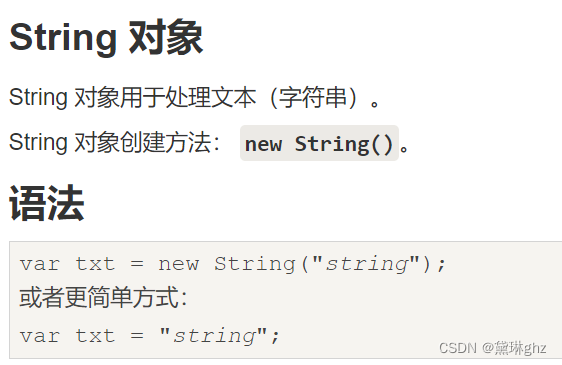JavaScript 字符串（String）对象的学习
0 0js内置对象:Array对象、Date对象、正则表达式对象、string对象
js内置对象:Array对象、Date对象、正则表达式对象、string对象
0 0ES6中Array对象的方法和扩展、string的扩展方法、数组的遍历。（含例题）

0 0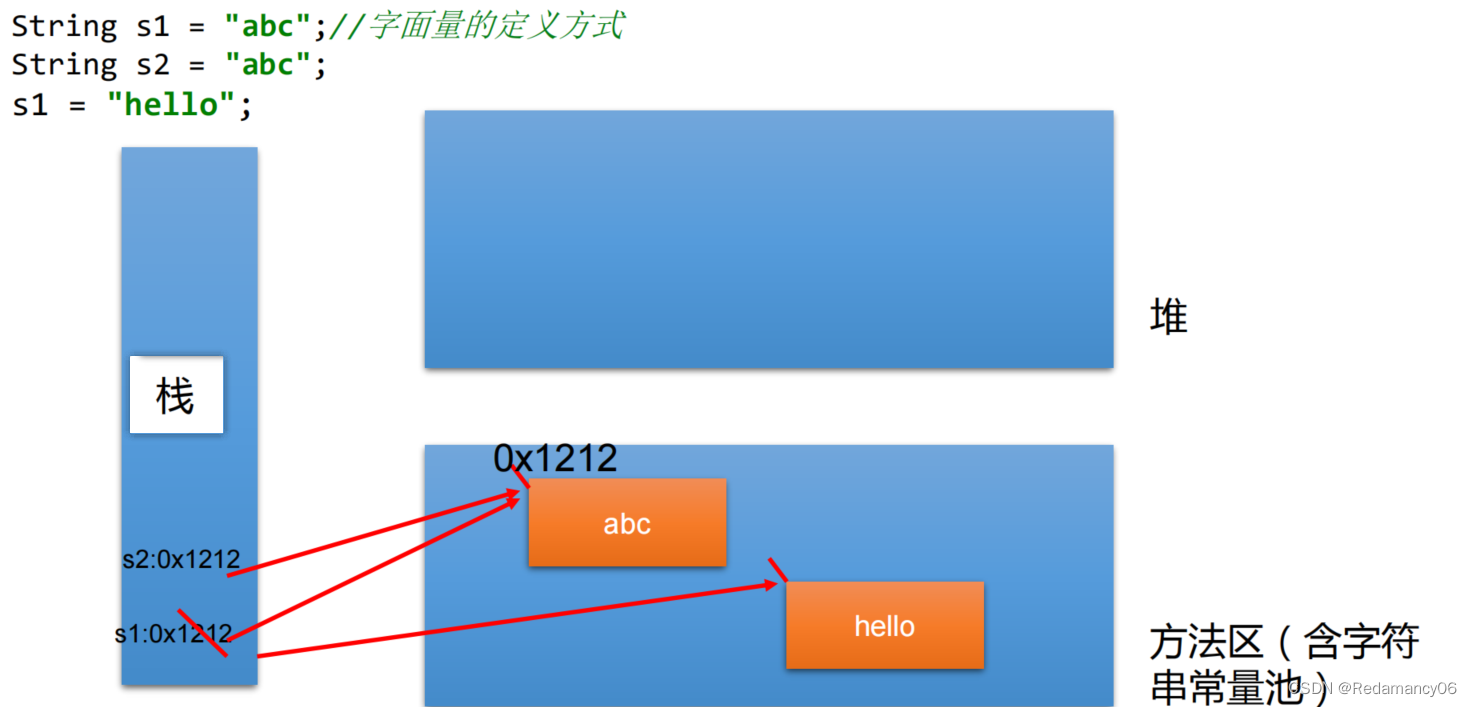Java常用类、String的特性、String对象的创建、字符串的特性、String使用陷阱、符串相关的类：String常用方法如toLowerCase（）、compareTo、substring
Java常用类、String的特性、String对象的创建、字符串的特性、String使用陷阱、符串相关的类：String常用方法如toLowerCase（）、compareTo、substring
0 0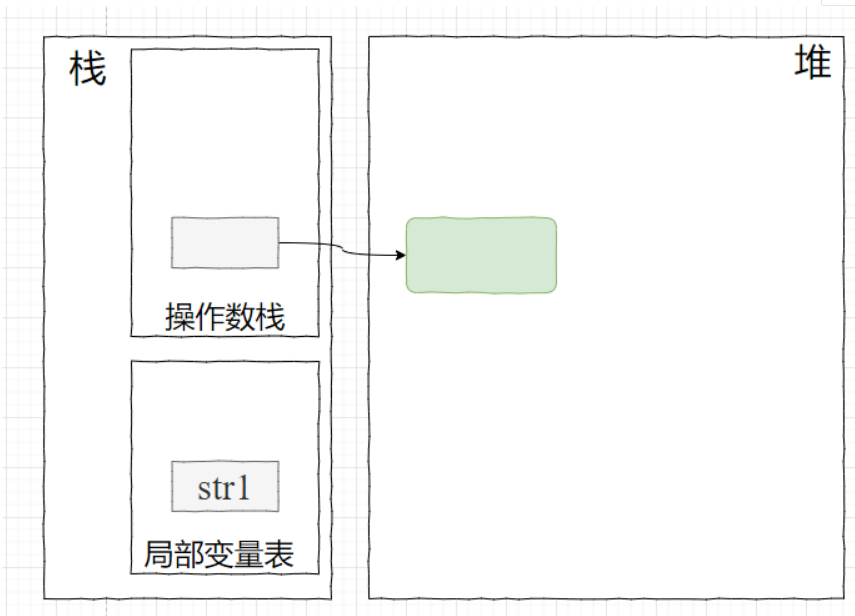0 023、String 对象
String对象是 JavaScript 原生提供的三个包装对象之一，用来生成字符串对象。
0 0

JavaScript入门与实战1896252

JavaScript 自学手册文档教程313565

Javascript中的对象

Javascript中的函数

JavaScript函数